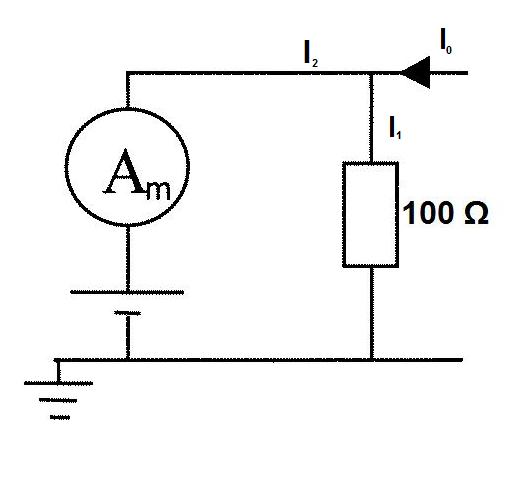#### You may also like### Overarch 2

Bricks are 20cm long and 10cm high. How high could an arch be built without mortar on a flat horizontal surface, to overhang by 1 metre? How big an overhang is it possible to make like this?### Stonehenge

Explain why, when moving heavy objects on rollers, the object moves twice as fast as the rollers. Try a similar experiment yourself.### Maximum Flow

Given the graph of a supply network and the maximum capacity for flow in each section find the maximum flow across the network.

# Battery Modelling

##### Age 16 to 18 Challenge Level:Part 1:

The ammeter will drop a voltage of 2V at 20A, it can therefore be modelled as a resistor of 0.1$\Omega$.

The $100\Omega$ resistor is in parallel with the series combination of the ammeter and battery.

$100 I_1 = (0.1x2) + (2x2) + 24$

$I_1 = 0.282 A$

Current Conservation:

$I_0 = I_1 + I_2$

$I_0 = 2 + 0.282 = 2.282 A$

Part 2:

Power supplied = Power consumed

Power Supplied = $(0.282^2 x100) + (2^2 x 0.1) + (2x2^2) + (2x24)$ = 64.35 W

Power dissipated by battery = IV = 2 x 24 = 48W

74.6 % of the power supllied is fed into the battery.

Part 3:

$I_0$ is unchanged, $I_0$ = 2.282A

Current conservation:

$I_0 = I_1 + I_2$

Voltage Conservation:

$100 I_1 = 24 + 2I_2$

Solving these two equations simultaneously we find $I_2 = \frac{I_0 - 0.24}{1.02} = 2.002 A$